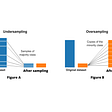# OOP + MachineLearning = Powerful

Being a data scientist is not easy and can be exhausting at times. There are so many facets of this field, keeping tab on each one of them could be tedious. For people who are starting with data science, python programming, or machine learning concepts, especially those who do not have a programming background, things can be much harder.

When I started out, even now to some extent, I struggle with OOP(Object Oriented Programming) concepts. It’s usefulness and effectiveness makes me want to learn it, but I always want some fun examples to grasp a concept. That is exactly what my intention here. I have to tried to use a simple example of linear regression to demonstrate the core concepts of OOP. So lets get started:

Lets try to get a dataset, and some prediction first:

`from numpy import arrayfrom numpy.linalg import invimport matplotlib.pyplot as pltdata = array([   [0.05, 0.12],   [0.18, 0.22],   [0.31, 0.35],   [0.42, 0.38],   [0.5, 0.49],   ])#separate out X and y and reshapeX = data[:,0]y = data[:,-1]X = X.reshape(-1,1)y = y.reshape(-1,1)#let's try to calculate coef using linear algebra, to predict the y #which is = coef*X (not including the intercept at this moment)coef_ = inv(X.T.dot(X)).dot(X.T).dot(y)yhat = X.dot(coef_)#Finally let's plot the dataplt.scatter(X, y)plt.plot(X, yhat, color='red')plt.xlabel('X')plt.ylabel('Y')plt.show()`

The above code leads to the following plot: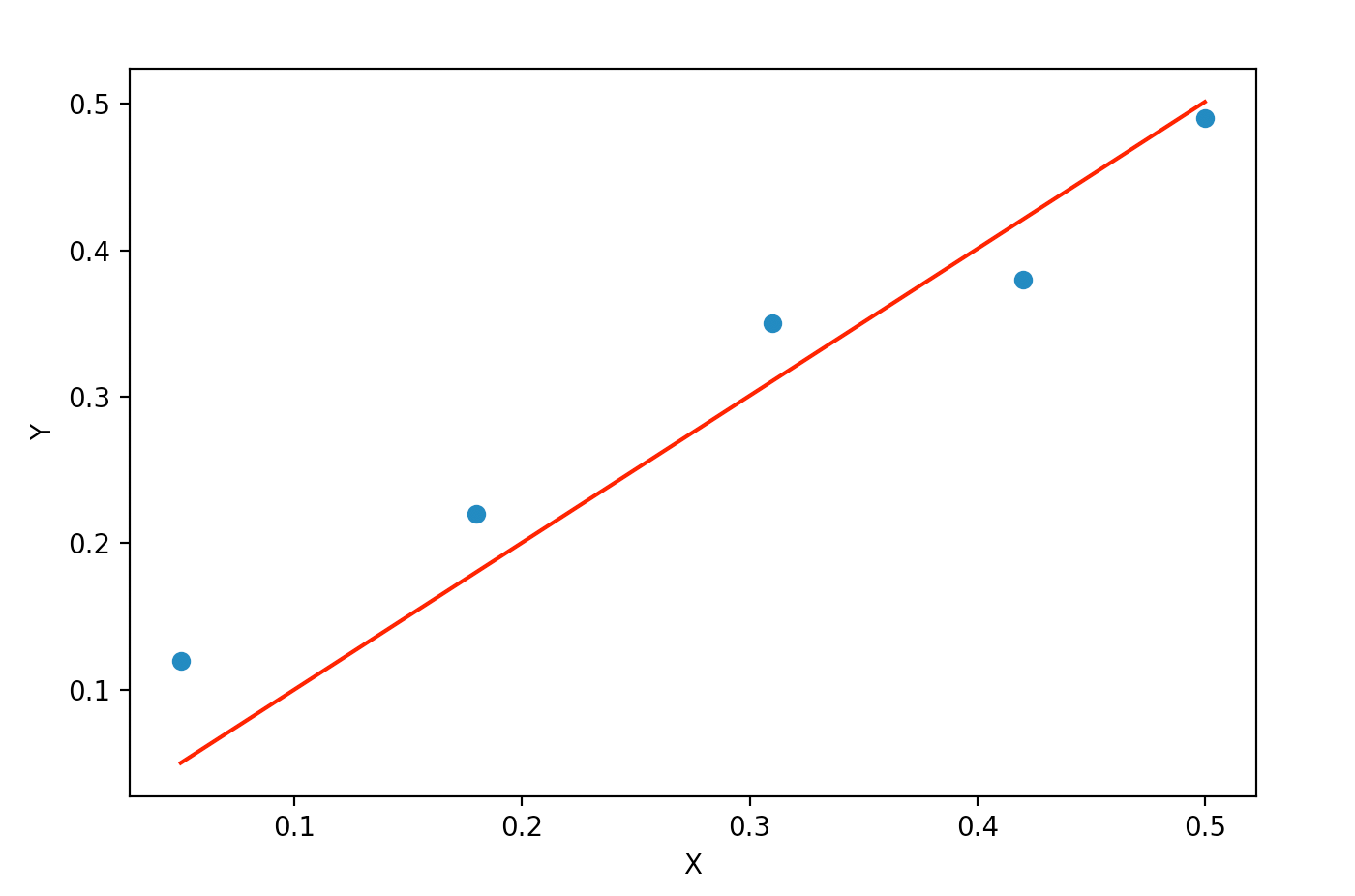where the scatter plot is for the actual data, and the line indicates the prediction

The whole point of adding this easy implementation of linear regression is that, now I am going to explore the OOP concept through the linear regression implementation above.

Let’s start by creating our class linear regression which we will write from scratch:

`import numpy as npfrom numpy import arrayfrom numpy.linalg import invimport matplotlib.pyplot as pltclass LinearRegression():    def __init__(self):       '''initializes the variables coef and pred'''        self.coef = None        self.pred = None    def fit(self,X,y):        '''calculate the coef ''        self.X = X        self.y = y        if len((self.X).shape) == 1:            self.X = (self.X).reshape(-1, 1)       self.coef=inv(self.X.T.dot(self.X)).dot(self.X.T).dot(self.y)    def predict(self):        '''predict the y values using the coef calculated above'''        if len((self.X).shape) == 1:            self.X = (self.X).reshape(-1, 1)        self.pred=  self.X.dot(self.coef)        return self.pred    def plt_prediction(self):        '''generates some plot'''        plt.scatter(self.X, self.y)        plt.plot(self.X, self.pred, color  = "red")        plt.show()`

__init__ : default constructor that gets called whenever we try to create an instance of the LinearRegression class. In this case, it will initialize two placeholders : coef and the pred which will have values later when we call the fit method and the predict method.

fit(X,y): this is a method which does the actual work of calculating the coef using the X and y values.

predict():this method when called, predicts the values and stores them in the pred variable initialed before.

plt_predict(): finally this method generates the same plot as shown above.

Now the fun part, lets make an instance and see the magic:

`mylinearreg = LinearRegression()mylinearreg.fit(X,y)print(mylinearreg.predict())output:[0.05011661 0.18041981 0.310723   0.42097955 0.50116613]`

Now, let’s create a base class from which my LinearRegression will be derived. What could I make my base class ??? a class called Metrics ??? we do need to evaluate our model, right???? Let’s do that:

`class Metrics:    def sse(self):        squared_errors = (self.y - self.pred) ** 2        self.sq_error_ = np.sum(squared_errors)        return self.sq_error_    def sst(self):        '''returns total sum of squared errors (actual vs avg(actual))'''        avg_y = np.mean(self.y)        squared_errors = (self.y - avg_y) ** 2        self.sst_ = np.sum(squared_errors)        return self.sst_    def r_squared(self):        '''returns calculated value of r^2'''        self.r_sq_ = 1 - self.sse() / self.sst()        return self.r_sq_class LinearRegression(Metrics):    def __init__(self):        self.coef = None        self.pred = None    def fit(self,X,y):        self.X = X        self.y = y        if len((self.X).shape) == 1:            self.X = (self.X).reshape(-1, 1)        self.coef = inv(self.X.T.dot(self.X)).dot(self.X.T).dot(self.y)    def predict(self):        if len((self.X).shape) == 1:            self.X = (self.X).reshape(-1, 1)        self.pred=  self.X.dot(self.coef)        return self.pred    def plt_prediction(self):        plt.scatter(self.X, self.y)        plt.plot(self.X, self.pred, color  = "red")        plt.show()`

Couple of points to observe, here :

1. my base class(“Metrics”) doesn’t have __init__ method, because the moment I make an instance of LinearRegression, it will automatically get all the methods defined in the base class(“Metrics”) and the base class now will use the __init__ method of the derived class(“LinearRegression”).

2. The moment I call the methods which reside in the base class, it will automatically take variables from the derived class.

Let’s make some calls now:

`mylinearreg = LinearRegression()mylinearreg.fit(X,y)print(mylinearreg.predict())print("The sse is: " , mylinearreg.r_squared())output: The sse is:  0.8820779000238227`

Isn’t this interesting. Now, we can add our customized metrics in the Metrics class and use them for other models, not just Linear Regression, by creating separate classes and making them all inherit from the base class. :)

Feel free to try this out with larger dataset and more complex methods, for instance adding a method that takes care of the gradient descent.

References:

https://machinelearningmastery.com/solve-linear-regression-using-linear-algebra/

--

--

--

## More from Analytics Vidhya

Analytics Vidhya is a community of Analytics and Data Science professionals. We are building the next-gen data science ecosystem https://www.analyticsvidhya.com

## Basic overview of some datatypes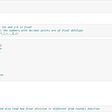## Plan a trip to Seattle at a low price by using Seattle AirBnB## Introduction to Big Data## Getting Started with Satellite Data Processing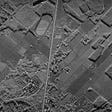## 2019 Syllabus: Criminal Justice Technology, Policy, & Law## CARTO + Matplotlib + Amazon AWS = popup joy!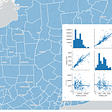## NAACOS Winter Bootcamp 2020## Dipanwita Mallick

I am working as a Senior Data Scientist at Hewlett Packard Enterprise. I love exploring new ideas and new places !! :)

## Machine learning — simple linear regression example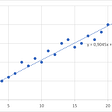## Data Wrangling Python## Python, the developer-oriented programming language## What is Imbalance data in Data science and how to Balance the same?